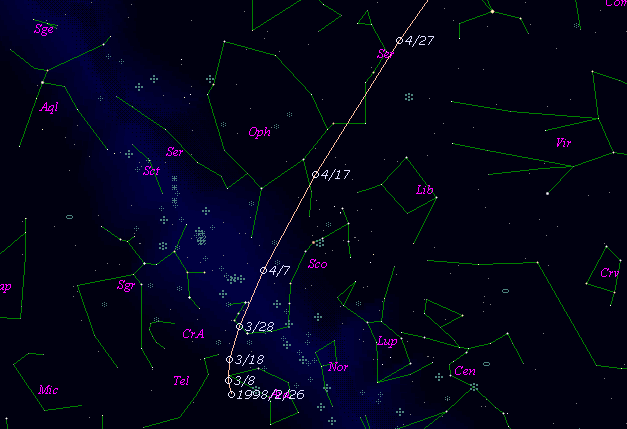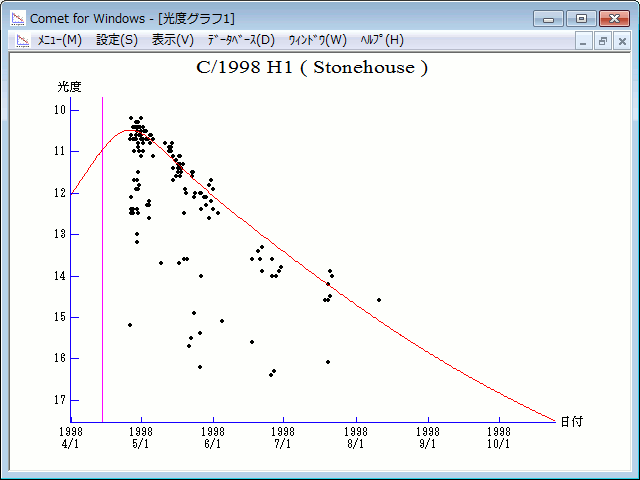# \$B%9%H!<%s%O%&%9WB@1(B

C/1998 H1 ( Stonehouse )###\$B%W%m%U%#!<%k(B

 \$BH/8+F|(B 1998\$BG/(B4\$B7n(B22\$BF|(B \$BH/8+8wEY(B 12\$BEy(B \$BH/8+ Patrick L. Stonehouse

###\$B###\$B50F;MWAG(B

```   The following improved orbital elements, by Kenji Muraoka are
from 352 observations  1998 Apr. 26  to  July 20, perturbations
by 9 Planets, Moon and 5 minor planets were taken into account.
The mean residual is +/- 0.68 arc seconds.

Epoch  =  1998 Apr. 17.0  TT       JDT = 2450920.5
T  =  1998 Apr. 14.42482       +/- 0.00074 (m.e.) TT
Peri. =    1.32431                +/- 0.00109
Node  =  222.10887                +/- 0.00029   (2000.0)
Incl. =  104.69219                +/- 0.00113
q  =    1.4872838              +/- 0.0000145 AU
e  =    0.9979059              +/- 0.0001737
1/a  =   +0.0014080              +/- 0.0001168 1/AU
( P  =  18927 years )
```

###\$B@1?^(B###\$B8wEYJQ2=(B

```        m1 = 10.0 + 5 log\$B&\$(B + 15.0 log r
```##### \$B50F;MWAG\$OB<2,7r<#;a\$N7W;;\$K\$h\$k\$b\$N\$G\$9!#(B \$B@1?^\$O(B StellaNavigator Ver.2.0 for Windows (\$B%"%9%H%m%"!<%D(B \$BJTCx(B / \$B%"%9%-!<=PHG6I4)(B) \$B\$G:n@.\$7\$?\$b\$N\$G\$9!#(B \$B8wEY%0%i%U\$O(BComet for Windows\$B\$G:n@.\$7\$?\$b\$N\$G\$9!#(B